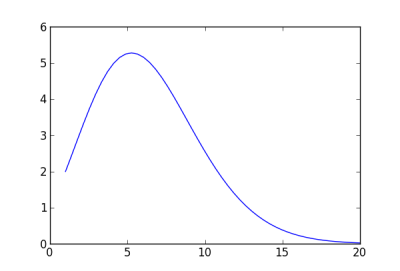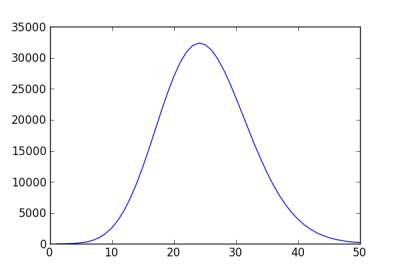# Dimension 5 isn’t so special

Lately I’ve been reading The Best Writing on Mathematics 2012. I’d like to present a alternative perspective on one of the articles.

In his article “An Adventure in the Nth Dimension,” Brian Hayes explores how in high dimensions, balls have surprisingly little volume. As the dimension n increases, the volume of a ball of radius 1 increases until n = 5. Then for larger n the volume steadily decreases. Hayes asks

What is it about five-dimensional space that allows a unit 5-ball to spread out more expansively than any other n-ball?

He says that it all has to do with the value of π and that if π were different, the unit ball would have its maximum value for a different dimension n. While that is true, it seems odd to speculate about changing the value of π. It seems much more natural to speculate about changing the radius of the balls.

The volume of a ball of radius r in dimension n isIf we fix r at 1 and let n vary, we get a curve like this:But for different values of r, the plot will have its maximum at different values of n. For example, here is the curve for balls of radius 2:Let’s think of n in our volume formula as a continuous variable so we can differentiate with respect to n. It turns out to be more convenient to work with the logarithm of the volume. This makes no difference: the logarithm of a function takes on its maximum exactly where the original function does since log is an increasing function.We can tell from this equation that volume (eventually) decreases as a function of n because ψ is an unbounded increasing function. The derivative has a unique zero, and we can move the location of that zero out by increasing r. So for any dimension n, we can solve for a value of r such that a ball of radius r has its maximum volume in that dimension:Related: High dimensional integration

## 14 thoughts on “Dimension 5 isn’t so special”

1. Does not compute. V(n,r) is [length^n]. Comparing length to area, area to volume, and so forth. Does it make sense?

What makes sense is how much smaller is the sphere w.r.t. the cube in the same dimension. And to tell that it’s better to deal with unit-cube, i.e. r=0.5 and n in Z, n > 0, then V(n,r=0.5) is decreasing monotonically. That is the higher n, the lower fraction of volume we can fill with spheres.

Can you give an interpretation of V(n,r) for a real n?

2. @S. Just so, those plots are comparing meters with liters.

3. That last formula doesn’t seem right. It doesn’t give something close to 1 for n=5, or something close to 2 for n=23.

4. Scott Ogawa

This is super interesting. Though something smells fishy. You are comparing the volumes across dimensions, yet this comparison depends on some arbitrary unit of measurement.

In other words, is a circle or a sphere (each with the same radius) “bigger”? If the answer depends on whether we use inches or centimeters, then that is not a well-posed question. Just because the definition “volume” has been generalized across dimensions does not mean we can do such comparisons.

5. Yes, it is fishy. If you don’t look at the volume relative to some other volume, you can get different results for different r’s.

6. DavidC

Along the same lines as the other comments: I’d say it’s NOT about the radius of the ball, but rather the units we use.

7. Dave Tate

Several of these results can be found in Steven Krantz’s article at http://www.maa.org/joma/volume7/krantz/higher.pdf, along with the very cool result that, in the limit as N goes to infinity, all of the volume of the N-dimensional unit ball lies arbitrarily close to the surface of the ball.

8. I think it’s intuitive:

A ball with radius 1 in n=2 IS a lot smaller than a ball with r=1 in n=100.
In order for the squared coordinates to add up to 1, a lot of them have to be small in the high-dimensional case.

One could imagine a process whereby we extrude a line 1 unit on each side, getting a square. They you have to cut a bit off to get a circle. Then you extrude it into a cylinder. To get a unit sphere, you again need to cut a bit off. Etc.

9. Charles Karney

It’s obvious that the ball is largest when the dimension is 1 and
decreases in volume with increasing dimension. Just evaluate the volume
of the ball with fits inside a unit n-dimensional cube (i.e., r = 1/2).

On the contrary, it’s obvious that the ball is smallest when the
dimension is 1 and increases in volume with increasing dimension. Just
evaluate the volume of the ball which just surrounds a unit
n-dimensional cube (i.e., r = sqrt(n)/2).

Bottom line is it all depends on what you are comparing. The only thing
you can be sure about is that the volume of a zero-dimensional ball is
always 1.

10. Your last formula has two typos. It should be
r=exp((1/2)(psi(n/2+1)-log(pi)))
or simply
r=sqrt(e^psi(n/2+1)/pi)

11. Veky: Thanks. I’ve updated the post.

12. This isn’t just about a choice of units. Imagine that you have a set of cubes in different dimensions, each containing the largest possible sphere. Now imagine choosing random points in the box and compare the odds of those points also being within the sphere. Your odds are greatest in dimension 5. (Actually in the fractional dimension 5.2569464147072722). I still don’t really have a sense of why there is a local maximum in this curve.

13. Charles Karney

Melinda, the way you posed the problem, the odds are maximum when the dimension is 1 and fall off monotonically from there. (See my comment above.)

14. I see your point. I guess it really is about units. Still, this is a very unexpected curve even if we are comparing apples with oranges. Is there an intuitive way of seeing why this curve peaks near dimension 5?